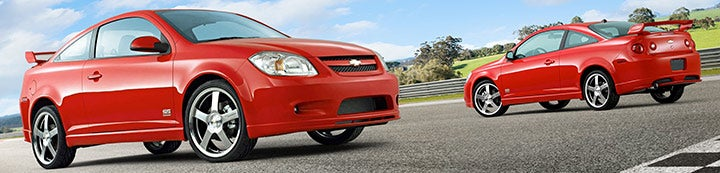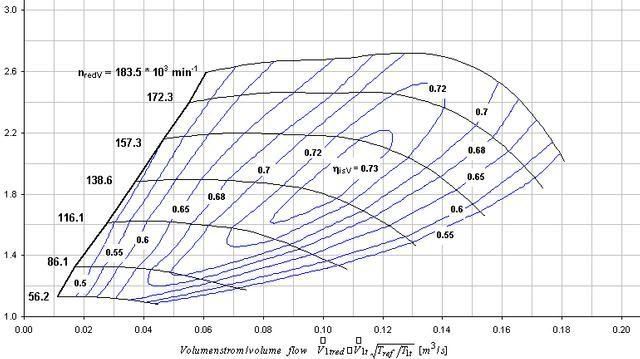1 - 3 of 3 Posts

#### Leveecius

·
##### Levee
Joined
·
3,210 Posts
Ok, so in this topic I am going to do my best to explain to you how to calculate and read compressor maps to help find the perfect sized turbo for the application that you're looking to put it into. This WILL get technical, so if you get lost, that's OK, that's what this is for, it's to help you learn to read them, as well as better your math skills lol. So with that being said, lets move on shall we? In all examples, we will be using a 4 stroke, 4 cylinder car with a 2.0L (122cid) displacement. Sound good? Ok, here we go:

First we need to calculate the engine air flow rate (CFM). The formula for this is:

CFM = L x RPM x VE x Pr/5660

Where L = engine capacity in liters
RPM = maximum engine speed (we'll adjust this later)
VE = engine volumetric efficiency. From A. Graham Bell's book Forced Induction Performance Tuning some good values for VE are:
Stock 2-valve = 85%
Stock 4-valve = 90%
Street modified = 93%
Competition = 105%
Pr = pressure ratio

To calculate the pressure ratio you need to know what boost pressure you want to run and then plug that into the following formula:

Pr = 14.7 + Boost/14.7

So - let's plug in some numbers and then apply them to the compressor maps. Say we want to run 18psi of boost. The pressure ratio comes out to be (14.7 + 18 ) / 14.7 = 2.22
Now lets calculate airflow. I think it's best to calculate airflow at at least two different RPM points. For our example, let's say we want to have full boost by half of max RPM. Redline on the Cobalt (from the factory) is 6700RPM. So we'll calculate airflow for 3350RPM and 6700RPM, and then see which map works out best for these values. We'll choose 90% for volumetric efficiency (VE).
For 670000RPM:
CFM = (2.0 x 6700 x 90 x 2.22) / 5660 = 473.02 or 33.1lb/min. To convert this value to lb/min take CFM and divide by 14.27.
For 3600RPM:
CFM = (2.0 x 3350 x 90 x 2.22) / 5660 = 236.5 or 16.5 lb/min. As a side note, since half the RPM will result in half the airflow, 236.5 is indeed half of 473.02

Now with all that being said, how does that help you on the compressor maps? Let me explain a compressor map first:Now, as you can see there are a LOT of numbers here, we won't go into all of them because quite frankly, I'm not 100% sure what some of them actually mean lol. 99% of the compressor maps have the numbers on the left of the graph, and numbers running along the bottom. So let me explain these numbers.

The numbers on the left are in psig (Pounds per Square Inch Gauge). Psia (Pounds per Square Inch Absolute) is your atmospheric pressure (the perfect psia is 0, or -14.7psig). Now the absolute pressure at sea level is about 14.7psi. So if your boost/vacuum level is 0, you have perfect psia and are pulling in 14.7psi, and anything else on top of that is your boost pressure. So lets say for example you are running 18lbs/psi of boost on your turbo, you would use the pressure ratio equation (14.7+ Boost/14.7) which equates to the 2.2 on the map. Really simple huh? that is how you graph how far up you're running as far as boost. *Now for something a little off topic, think of this. If it is 14.7psi at sea level and you run 18 boost, you are actually getting 32.7 psi of pressure.Now the numbers on the bottom are the air flow numbers. Most graphs are written in either CFM's or lbs/min. THIS particular graph is written in Cubic Meters/Second. Now converting is is easy but you need a calculator. So here is how you convert it to CFM's

1 Cubic Meter/Second = 2119 Cubic Feet/Meter. So to convert this you will need to take 2119 and multiply it by the bottom numbers (for example .10 = 211.9 (or 212 if you want to round up as most do)). *I will post a graph later of the converted numbers unless someone does it before me*

Now to get from Cubic Feet/Meters to lbs/min, you will simply multiply CFM by .0807. (For example again - .10 cM/s x 2119 x .0807= 17.10 lbs/min air flow). I will also add a chart that shows this.

Now Air flow is very important when looking at power of cars. It can actually tell you quite a bit about your engine regardless of boost or not. I will get into that if and only if people ask me to get into that in this thread.

Now back to the numbers. In the graph you will see "rings/layers" on the graph with numbers with them (.5, .6 ect) These numbers represent the efficiency ranges of the turbo for certain boost and air flow points. This is where everything starts coming together. If you plot our point in the example (18psi/ 2.2psig), our engines at 90% VE are running at the 212cfm's, (using simple geometry) you get the point where you are between .72 and .7, so you are at .71, which turns into 71% Efficiencly.

Now there are some graphs that have numbers all the way to the right and they usually are 6 digits long. Those are the compressor RPM numbers, basically telling you about how fast that turbo is spinning.

Now another really important thing to look at on these graphs is the bolded line on the left of the compressor map that follows the left side of the graph. This is your surge line. It is VERY bad to have a point to the LEFT of this line. Just so you know lol.

I was doing a little more than the usual and decided to get this done for you guys already. Here is a CFM/RPM/Boost table that I calculated (with a calculator mind you), this will help you show you EXACTLY how much air your engine will flow at 90% VE with 18psi of boost to 6700rpms. This chart will help you plot your points on a compressor map to see what turbo is right for you.

That being said here is a link to the map for a 2.0:
http://cybrina.mine.nu:8080/WebModule2/compcalc?size=2.0&ve=90&boost=18&maxrpm=6700&rpmstep=50&Submit=Submit

2.2:
http://cybrina.mine.nu:8080/WebModule2/compcalc?size=2.2&ve=90&boost=18&maxrpm=6700&rpmstep=50&Submit=Submit

2.4:
http://cybrina.mine.nu:8080/WebModule2/compcalc?size=2.4&ve=90&boost=18&maxrpm=6700&rpmstep=50&Submit=Submit

Any questions as of yet?

#### Leveecius

·
##### Levee
Joined
·
3,210 Posts
Discussion Starter
Also something to keep in mind, the higher the RPM of the engine to more boost drop you will get if you start going out of your efficiency range, or breathing range of your turbo.

·